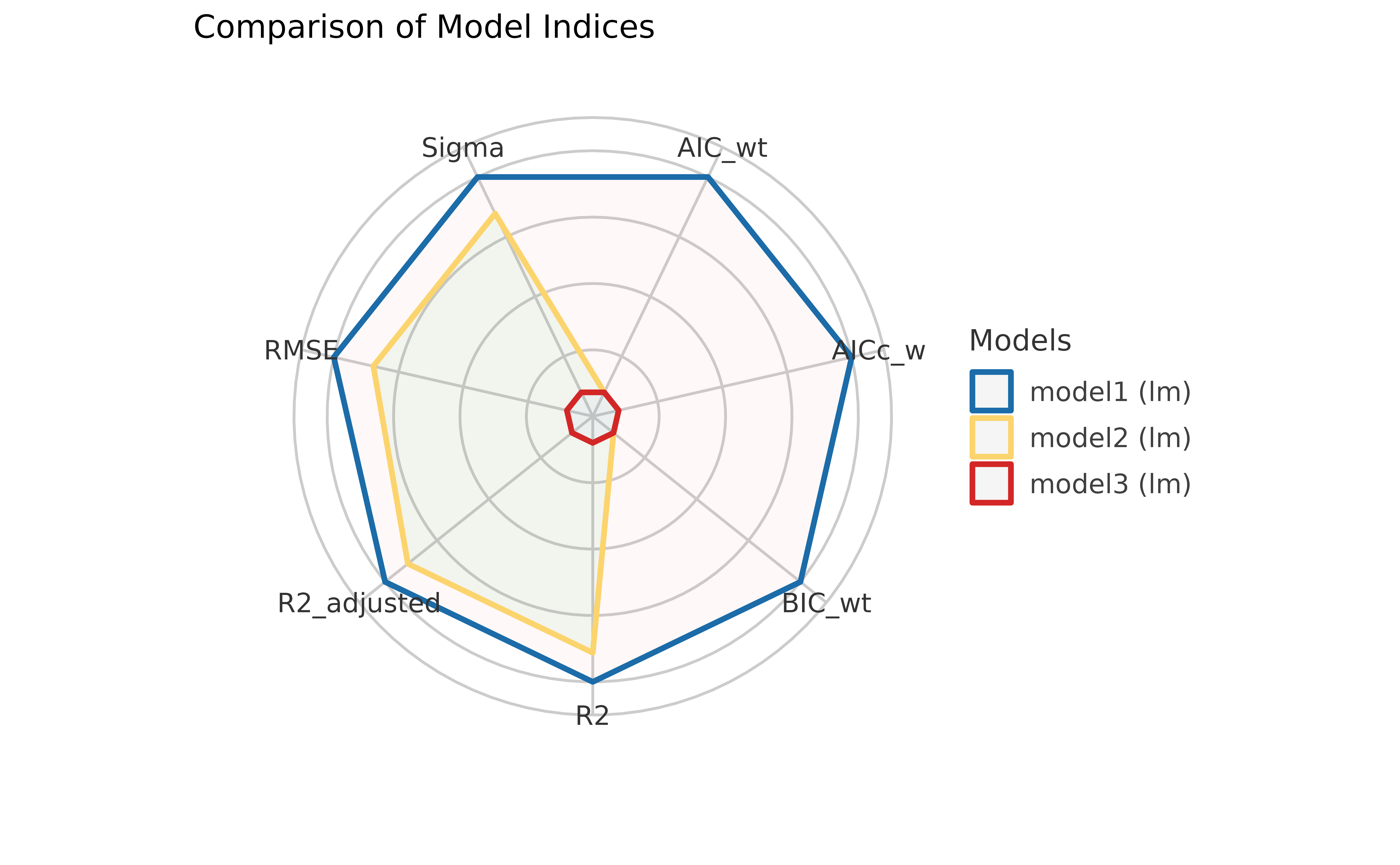## Comparing vs. Testing

Let’s imagine that we are interested in explaining the variability in the Sepal.Length using 3 different predictors. For that, we can build 3 linear models.

model1 <- lm(Sepal.Length ~ Petal.Length, data = iris)
model2 <- lm(Sepal.Length ~ Petal.Width, data = iris)
model3 <- lm(Sepal.Length ~ Sepal.Width, data = iris)

### Comparing Indices of Model Performance

The eponymous function from the package, performance(), can be used to compute different indices of performance (an umbrella term for indices of fit).

library(performance)
library(insight)
library(magrittr) # for pipe operator

# we will use print_md function to display a well-formatted table
performance(model1) %>%
print_md()
Indices of model performance
AIC BIC R2 R2 (adj.) RMSE Sigma
160.04 169.07 0.76 0.76 0.40 0.41

But for multiple models, one can obtain a useful table to compare these indices at a glance using the compare_performance() function.

compare_performance(model1, model2, model3) %>%
print_md()
Comparison of Model Performance Indices
Name Model AIC AIC weights BIC BIC weights R2 R2 (adj.) RMSE Sigma
model1 lm 160.04 1.000 169.07 1.000 0.76 0.76 0.40 0.41
model2 lm 208.22 < 0.001 217.25 < 0.001 0.67 0.67 0.47 0.48
model3 lm 371.99 < 0.001 381.02 < 0.001 0.01 7.16e-03 0.82 0.83

If you remember your stats lessons, while comparing different model fits, you would like to choose a model that has a high $$R^2$$ value (a measure of how much variance is explained by predictors), low AIC and BIC values, and low root mean squared error (RMSE). Based on these criteria, we can immediately see that model1 has the best fit.

If you don’t like looking at tables, you can also plot them using a plotting method supported in see package:

library(see)

plot(compare_performance(model1, model2, model3))### Testing Models

While comparing these indices is often useful, making a decision (for instance, which model to keep or drop) can often be hard, as the indices can give conflicting suggestions. Additionally, it is sometimes unclear which index to favour in the given context.

This is one of the reason why tests are useful, as they facilitate decisions via (infamous) “significance” indices, like p-values (in frequentist framework) or Bayes Factors (in Bayesian framework).

test_performance(model1, model2, model3) %>%
print_md()
Name Model BF Omega2 p (Omega2) LR p (LR)
model1 lm
model2 lm < 0.001 0.19 < .001 4.57 < .001
model3 lm < 0.001 0.56 < .001 11.52 < .001

Each model is compared to model1.

However, these tests also have strong limitations and shortcomings, and cannot be used as the one criterion to rule them all!

### Experimenting

Although we have shown here examples only with simple linear models, we will highly encourage you to try these functions out with models of your choosing. For example, these functions work with mixed-effects regression models, Bayesian regression models, etc.

To demonstrate this, we will run Bayesian versions of linear regression models we just compared:

library(rstanarm)

model1 <- stan_glm(Sepal.Length ~ Petal.Length, data = iris, refresh = 0)
model2 <- stan_glm(Sepal.Length ~ Petal.Width, data = iris, refresh = 0)
model3 <- stan_glm(Sepal.Length ~ Sepal.Width, data = iris, refresh = 0)

compare_performance(model1, model2, model3) %>%
print_md()
Comparison of Model Performance Indices
Name Model ELPD ELPD_SE LOOIC LOOIC weights LOOIC_SE WAIC WAIC weights R2 R2 (adj.) RMSE Sigma
model1 stanreg -80.02 8.35 160.04 1.000 16.70 160.03 1.000 0.76 0.76 0.40 0.41
model2 stanreg -104.22 9.26 208.43 < 0.001 18.52 208.42 < 0.001 0.67 0.66 0.47 0.48
model3 stanreg -185.70 7.88 371.41 < 0.001 15.76 371.39 < 0.001 0.01 -1.71e-03 0.82 0.83

Note that, since these are Bayesian regression models, the function automatically picked up the appropriate indices to compare!

If you are unfamiliar with some of these, explore more here.

Now it’s your turn to play! :)